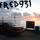General QuestionCan I create a spiral with Roundline in Rainmeter?

Asked by Fred931 (9409) May 5th, 2013

Visualize the pupil of an eye and how it contracts and expands. That is the effect I am trying to generate in a skin using the roundline meter. The idea is to make the roundline complete multiple rotations, and add some sort of equation to the angle configuration so that there is a very slight spiral as the completions are made.

This is my first skin, so not only are my math skills not good enough to understand this, but I also am still struggling with the programming and all. Is it possible to write an equation for this in the program? What would that equation be? (radius is 66pix, startangle is 270*) Is there a better way?

Observing members: 0Composing members: 0or